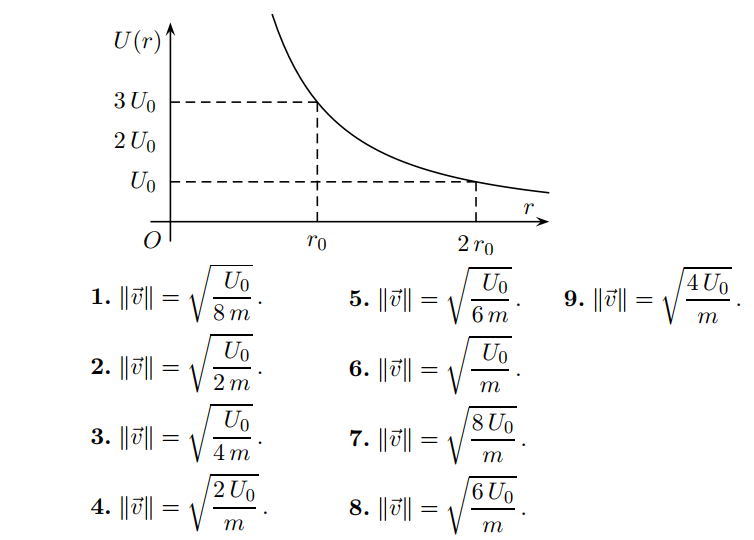# Problem: The following graph represents a hypothetical potential energy curve for a particle of mass m.If the particle is released from rest at position r 0, its speed ||→v|| at position 2r0 is most nearly

###### FREE Expert Solution
88% (250 ratings)
###### Problem Details

The following graph represents a hypothetical potential energy curve for a particle of mass m.

If the particle is released from rest at position r 0, its speed ||v|| at position 2r0 is most nearlyFrequently Asked Questions

What scientific concept do you need to know in order to solve this problem?

Our tutors have indicated that to solve this problem you will need to apply the Force & Potential Energy concept. You can view video lessons to learn Force & Potential Energy. Or if you need more Force & Potential Energy practice, you can also practice Force & Potential Energy practice problems.

How long does this problem take to solve?

Our expert Physics tutor, Juan took 1 minute and 54 seconds to solve this problem. You can follow their steps in the video explanation above.

What professor is this problem relevant for?

Based on our data, we think this problem is relevant for Professor Perry's class at TEXAS.